# Math in Focus Grade 3 Chapter 4 Answer Key Subtraction up to 10,000

Go through the Math in Focus Grade 3 Workbook Answer Key Chapter 4 Subtraction up to 10,000 to finish your assignments.

## Math in Focus Grade 3 Chapter 4 Answer Key Subtraction up to 10,000

Challenging Practice
Fill in the blanks in each number sentence. Use the numbers in the box.

Question 1.
The difference between two numbers is 42.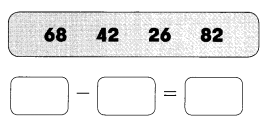The difference between two numbers is 42 are 68 and 26.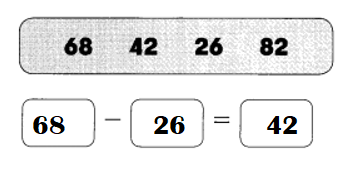Explanation:
Difference:
1. 68 – 42 = 26.       68 – 26 = 42.       82 – 68 = 14.
2. 42 – 26 = 26.
3. 26
4. 82 – 68 = 14.        82 – 42 = 40.       82 – 26 = 56.

Question 2.
The difference between two numbers is 280.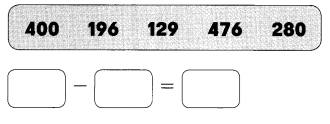The difference between two numbers is 280 are 476 and 196.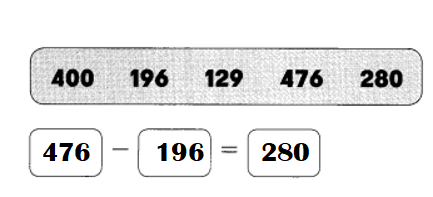Explanation:
Difference:
1. 400 – 196 = 204.         400 – 129 = 271.           400 – 280 = 120.
2. 196 – 129 = 67.
3. 129
4. 476 – 400 = 76.       476 – 196 = 280.       476 – 129 = 347.        476 – 280 = 196.
5. 280 – 196 =          280 – 129 = 151.

Fill in the missing numbers.
Question 3.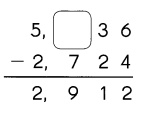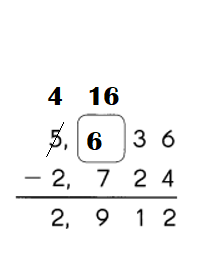Explanation:
Ones place number: 6 – 4 = 2.
Tens place number: 3 – 2 = 1.
Hundreds place number: ?? – 7 = 9
=> ?? = 9 + 7
=> ?? = 16(10 + 6).
Thousand place number: 5 – 1 = 4.
=> 4 – 2 = 2.

Question 4.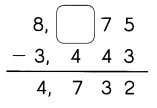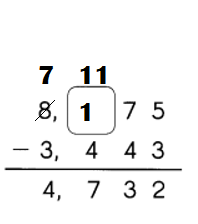Explanation:
Ones place number: 5 – 3 = 2.
Tens place number: 7 – 4 = 3.
Hundreds place number: ?? – 4 = 7
=> ?? = 7 + 4
=> ?? = 11(1 + 10).
Thousand place number: 8 – 1 = 7.
=> 7 – 3 = 4.

Fill in the missing numbers.

Question 5.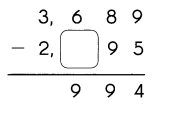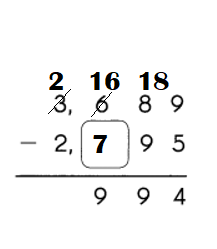Explanation:
Ones place number: 9 – 5 = 4.
Tens place number: 8 – 9 = 9.
=> (10 + 8) – 9 = 9.
Hundreds place number: 6 – ?? = 9.
=> ?? = 9 + 6
=> ?? = 15(5 + 10).
=> 15 – ?? = 9
=> ?? = 15 – 9
=> ?? = 6.
Thousand place number:
3 – 1 = 2.
=> 2 – 2 = 0.

Question 6.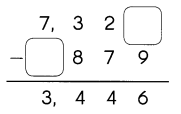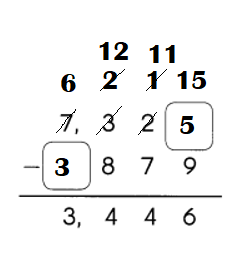Explanation:
Ones place number: ?? – 9 = 6.
=> ?? = 6 + 9
=> ?? = 15. 15 = (10 + 5)
Tens place number: 2 – 1 = 1.
=> 1 – 7 = 4.(NO)
=> 11 (10 + 1) – 7 = 4.
Hundreds place number: 3 – 1 = 2.
=> 2 – 8 = 4(NO)
=> (2 + 10 )12 – 8 = 4.
Thousand place number: 7 – 1 = 6.
=> 6 – 3 = 3.

Solve. Use the digits to make 4-digit numbers. Show your work. Do not begin any number with ‘0’.

Question 7.
Subtract the least 4-digit number from the greatest 4-digit number.
_____ – ____ = ____
9999 – 1000
= 8999.

Explanation:
Least 4-digit number = 1000.
Greatest 4-digit number = 9999.
Difference:
Greatest 4-digit number – Least 4-digit number
= 9999 – 1000
= 8999.

Solve.
Question 8.
Write a number greater than 5,632 using the digits 0, 1, 4, 7. Then subtract 5,632 from the number.
____ – ____ = _____
7410 – 5632
= 1778.

Explanation:
Number greater than 5,632 using the digits 0, 1, 4, 7.
Number greater than 5,632 = 7410.
Then subtract 5,632 from the number.
=> Difference:
=> Number greater than 5,632 – 5,632
=> 7410 – 5632
=> 1778.

Problem Solving
Question 1.
The difference between two numbers is 100. One number is more than 90 but less than 100. The other number is between 190 and 200. What are the two possible numbers?
____ and ____
The two possible numbers are 199 and 99.

Explanation:
Let the two numbers be X and Y.
The difference between two numbers is 100.
=> X – Y = 100.
One number is more than 90 but less than 100.
=> One Number = 99.
The other number is between 190 and 200.
=> Other number = 199.
Difference:
199 – 99 = 100.

Question 2.
Lilian went shopping with $1,000. She saw five items on display in a shop window. After buying two items, she had$732 left. Which two items did she buy?
___ and ____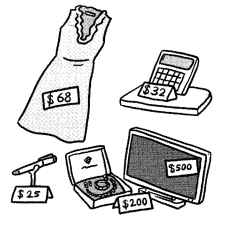Two items she bought are frock and necklace set.

Explanation:
Cost of frock = $68. Cost of calculator =$32.
Cost of mick = $25. Cost of necklace set =$200.
Cost of Television = $500. Amount of money Lilian went shopping =$1000.
After buying two items, she had $732 left. Amount of money spent = Amount of money Lilian went shopping – Amount of money left after buying 2 items =$1000 – $732 =$268. ($68 +$200) (Cost of frock + Cost of necklace set)

Solve.
Question 3.
Nick and Isaac are at a school fair.
They want to collect points to exchange for these prizes.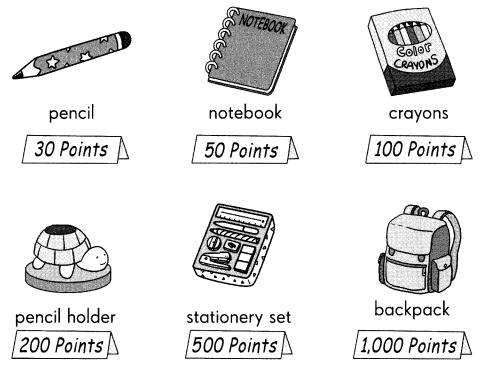At the fair games, Nick has 215 points and Isaac has 78 points. They combine their points to exchange for three prizes.
What are the two sets of three prizes they can get?

a. ________________
Three prizes = 2(Pencils) and Pencil holder.

Explanation:
Points of Nick has = 215.
Points of Isaac has = 78.
Total combined points of Nick and Isaac = Points of Nick has  + Points of Isaac has
= 215 + 78
= 293.
Points of pencil = 30.
Points of pencil holder = 200.
Total points of three items  = 2(Points of pencil) + Points of pencil holder
= 2(30) + 200
= 60 + 200
= 260.

b. ________________
Three things are Pencil, Notebook and pencil holder.

Explanation:
Points of Nick has = 215.
Points of Isaac has = 78.
Total combined points of Nick and Isaac = Points of Nick has  + Points of Isaac has
= 215 + 78
= 293.
Points of pencil holder = 200.
Points of Pencil  = 30.
Points of Notebook = 50.
Total points of three items  = Points of pencil holder + Points of Pencil  + Points of Notebook
= 200 + 30 + 50
= 230 + 50
= 280.